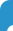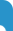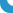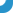## Members of Academic Board - Abbas NajatiSections ListDr Abbas Najati | Back to CV page | [Print version]Published articles ISI Journals (1) A. Najati, The generalized Hyers–Ulam–Rassias stability of a cubic functional equation, Turkish Journal of Mathematics 31 (2007), 395– 408. (2) A. Najati and C. Park, Hyers–Ulam –Rassias stability of homomorphisms in quasi-Banach algebras associated to the Pexiderized Cauchy functional equation, J. Math. Anal. Appl. 335 (2007), 763–778. (3) C. Park and A. Najati, Homomorphisms and derivations in C*-algebras, Abstract and Applied Analysis (2007) Article ID 80630, 12 pages. (4) A. Najati and M. B. Moghimi, Stability of a functional equation deriving from quadratic and additive functions in quasi–Banach spaces, J. Math. Anal. Appl. 337 (2008) 399–415. (5) Abbas Najati, Hyers–Ulam–Rassias stability of a cubic functional equation, Bull. Korean Math. Soc. 44 (2007), 825–840. (6) Abbas Najati, Hyers–Ulam stability of an n Apollonius type quadratic mapping, Bull. Belg. Math. Soc. Simon Stevin 14 (2007), 755–774. (7) A. Najati, On the stability of a quartic functional equation, J. Math. Anal. Appl. 340 (2008), 569–574. (8) A. Najati and A. Ranjbari, Stability of homomorphisms for a 3D Cauchy-Jensen type functional equation on C-ternary algebras, J. Math. Anal. Appl., 341 (2008), 62–79. (9) A. Najati and G. Zamani Eskandani, Stability of a mixed additive and cubic functional equation in quasi- Banach spaces, J. Math. Anal. Appl. 342 (2008), 1318-1331. (10) Abbas Najati and Choonkil Park, The Pexiderized Apollonius-Jensen type additive mapping and isomorphisms between C-algebras, J. Difference Equ. Appl. 14 (2008), 459–479. (11) Abbas Najati and Asghar Rahimi, A fixed point approach to the stability of a generalized Cauchy functional equation, Banach J. Math. Anal. 2 (2008), 105–112. (12) Abbas Najati and F. Moradlou, Stability of a quadratic functional equation in quasi Banach spaces, Bull. Korean Math. Soc. 45 (2008), 587–600. (13) Abbas Najati and A. Rahimi, Euler–Lagrange type cubic operators and their norms on X space, J. Inequal. Appl. (2008), Article ID 195137, 8 pages. (14) A. Najati and C. Park, On the Stability of an n–Dimensional Functional Equation Originating from Quadratic Forms, Taiwanese J. Math. 12 (2008), 1609–1624. (15) A. Najati and C. Park, On the stability of a cubic functional equation, Acta Mathematica Sinica 24 (2008), 1953–1964. (16) Abbas Najati, G. Zamani and C. Park, Stabiliy of homomorphisms and derivations in proper JCQ-triples associated to the pexideraized Cauchy type mapping, Bull. Korean Mah. Soc. 46 (2009), 45–60. (17) Abbas Najati and F. Moradlou, Stability of an Euler-Lagrange type cubic functional equation, Turkish J. Math. 33 (2009), 65–73. (18) M.S. Moslehian and Abbas Najati, An application of a fixed point theorem to a functional inequality, Fixed Point Theory, 10 (2009) No. 1, 141–149. (19) Abbas Najati and C. Park, Fixed points and stability of a generalized quadratic functional equation, J. Inequalities and Applications, (2009) Article ID 193035, 19 pages. (20) Abbas Najati, On a problem of C. Baak and M.S. Moslehian, Applied Mathematics Letters, 22 (2009), 658–660. (21) Abbas Najati, Jordan -derivation on Lie triple systems, Bull. Korean Math. Soc. 46 (2009), No. 3, 435–437. (22) Abbas Najati and A. Rahimi, Generalized frames in Hilbert spaces, Bull. Iranian Math. Soc. 35 (2009), No. 1, 97–109. (23) Abbas Najati, C. Park and J.R. Lee, Homomorphisms and derivations in C*-ternary algebras, Abst. Appl. Anal. (2009), Article ID 612392, 16 pages. (24) C. Park, W.-G. Park and Abbas Najati, Functional equations related to inner product spaces, Abstract and Applied Analysis, (2009), Article ID 907121, 11 pages. (25) Abbas najati, Generalized derivations on Lie triple systems, Result. Math. 54 (2009), 143–147. (26) M. Moghimi, A. Najati and C. Park, A fixed point approach to the stability of a quadratic functional equation in C-Algebras, Advances in Difference Equations, (2009), Article ID 256165, 10 pages. (27) M. Moghimi, A. Najati and C. Park, A functional inequality in restricted domains of Banach modules, Advances in Difference Equations, (2009), Article ID 973709, 14 pages. (28) Abbas Najati and C. Park, Stability of a generalized Euler-Lagrange type additive mapping and homomorphisms in C-Algebras, Advances in Difference Equations, (2009), Article ID 273165, 22 pages. (29) Abbas Najati, Homomorphisms in quasi-Banach algebras associated with a Pexiderized Cauchy–Jensen functional equation, Acta Mathematica Sinica, English Series, 25 (2009), No. 9, 1529–1542. (30) Abbas Najati and Th.M. Rassias, Stability of homomorphisms and derivations, Appl. Anal. Discrete Math. 3 (2009), 264–281. (31) Abbas Najati and Th.M. Rassias, Stability of a mixed functional equation in several variables on Banach modules, Nonlinear Analysis- Theory Methods and Applications 72 (2010), 1755–1767. (32) Abbas Najati and Th.M. Rassias, Pexider type operators and their norms in \$X_{lambda}\$ spaces, Czechoslovak Mathematical Journal, 59 (134) (2009), 1087--1094. (33) Abbas Najati and C. Park, Stability of homomorphisms and generalized derivations on Banach algebras, J. Inequal. Appl. (2009), Article ID 595439, 12 pages. (34) F. Moradlou, A. Najati and H. Vaezi, Stability of homomorphisms and derivations on \$C^*\$-ternary rings associated to an Euler-Lagrange type additive mapping,  Result.Math. 55 (2009), 469--486. (35) M. Eshaghi Gordji and Abbas Najati, Approximately \$J^*\$-homomorphisms: A fixed point approach, Journal of Geometry and Physics 60 (2010), 809--814. (36) Abbas Najati, On the completeness of normed spaces, Applied Mathematics Letters 23 (2010) 880--882. (37) Abbas Najati, On generalized Jordan derivations of Lie triple systems, Czechoslovak Mathematical Journal 60 (135) (2010), 541--547. (38) Abbas Najati and G. Zamani Eskandani, A fixed point method to the generalized stability of a mixed additive and quadratic functional equation in Banach modules,  Journal of Difference Equations and Applications, Vol. 16, No. 7 (2010), 773--788. (39) Abbas Najati, Jung-Rye Lee and C. Park, On a Cauchy--Jensen funtional inequality, Bull. Malaysian Math. Sci. Soc. (2) 33 (2) (2010), 253--263. (40) A. Ebadian, A. Najati and M. Eshaghi Gordji, On Approximate Additive–Quartic and Quadratic–Cubic Functional Equations in Two Variables on Abelian Groups,  Results Math. 58 (2010), 39--53. (41) A. Rahimi and A. Najati, A strong quadratic functional equation in \$C^*\$-algebras, Fixed Point Theory, 11 (2010), 361--368. (42) A. Najati and S.-M. Jung, Approximately quadratic mappings on restricted domains, Journal of Inequalities and Applications, (2010), Article ID503458, 10 pages. (43) A. Rahimi, A. Najati and J.-H. Bae, On the asymptoticity aspect of Hyers--Ulam stability of quadratic mappings, Journal of Inequalities and Applications, (2010), Article ID 454875, 14 pages. (44) Abbas Najati and Yeol Je Cho, Generalized Hyers--Ulam stability of the Pexiderized Cauchy functional equation in non-Archimedean spaces,  Fixed Point Theory and Applications, (2011), Article ID 309026, 11 Pages. (45) M.R. abdollahpour and A. Najati, Stability of linear differential equations of third order, Applied Mathematics Letters,  Volume 24, Issue 11, November (2011), Pages 1827--1830. (46)  M.R. abdollahpour and A. Najati, Approximation of the inverse \$G\$-frame operator, Proceedings Mathematical Sciences, Volume 121, Number 2, (2011), Pages 143--154. (47)  M. Eshaghi Gordji, A. Najati and A. Ebadian, Stability and superstability of Jordan homomorphisms and Jordan derivations on Banach algebras and \$C^*\$-algebras: A fixed point approach, Acta Mathematica Scientia, Volume 31, Issue 5, September (2011), Pages 1911--1922. (48) Abbas Najati, Jung Im Kang and Yeol Je Cho, Local stability of the Pexiderized Cauchy and Jensen's equations in fuzzy spaces, Journal of Inequalities and Applications (2011), 2011:78, 8 pages. (49) Abbas Najati, M. R. Abdollahpour and Gwang Hui Kim, On Pexider Differences in Topological Vector Spaces, Abstract and Applied Analysis (2011), Article ID 370104, 10 pages. (50) M. R. Abdollahpour and A. Najati, Besselian \$g\$-frames and near \$g\$-Riesz bases, Appl. Anal. Discrete Math. 5 (2011), 259--270. (51) M. Eshaghi Gordji,H. Khodaei and A. Najati,  Approximation of cubic mappings with \$n\$-variables in \$beta\$-normed left Jordan modules on Banach algebras,  Bull. Korean Math. Soc. 48 (2011),  1063--1078. (52) C. Park, A. Najati and S.Y. Jang, Fixed points and fuzzy stability of an addiive-quadratic functional equation, J. Computational Analysis and Applications, Vol. 15 (2013), 452--462. (53) Abbas Najati, M. Mohammadi Saem and Jae-H. Bae, Generalized Dunkl-Williams inequality in 2-inner product spaces, Journal of Inequalities and Applications, 2013: 36 (2013) 8 pages. (54) Mohammad Reza Abdollahpour, Abbas Najati, Choonkil Park, Themistocles M Rassias and Dong You Shin, Approximate perfect differential equations of second order,  Advances in Difference Equations , 2012:225 (2012) 5 pages. ------------------------------------------------------------------------------------------------------------------------------------------------------------------------------------------------------------------------------------------------ (1) A. Rahimi, A. Najati and Y. N. Dehghan,  Continuous Frames in Hilbert Spaces, Methods of Functional Analysis and Topology 12 (2006), 170--182. (2) Abbas Najati, Stability of homomorphisms on \$JB^*\$-triples associated to a Cauchy-Jensen type functional equation, J. Math. Inequal. 1 (2007), 83--103. (3) Abbas Najati, On the stability of homomorphisms in quasi-Banach algebras associated to the Pexiderized Jensen functional equation, J. Math. Inequal. 1 (2007), 205--223. (4) A. Najati and M. H. Faroughi, \$p\$-frames of subspaces in separable Hilbert spaces, Southeast Asian Bulletin of Mathematics . 31 (2007), 713--726. (5) A. Najati and A. Ranjbari,  On homomorphisms between \$C^*\$-ternary algebras, J. Math. Ineq. 3 (2007) 387--407. (6) A. Najati, A. Rahimi and M.H. Faroughi, Continuous and discrete frames of subspaces in Hilbert spaces, Southeast Asian Bull. Math. 32 (2008) 305-324. (7) M.H. Faroughi and A. Najati, Ultra Bessel sequences in Hilbert spaces, Southeast Asian Bull. Math. 32 (2008) 425--436. (8) Abbas Najati, Approximation of g-frames in Hilbert spaces, African Diaspora J. Math.  6 (2008), 137--145. (9) Abbas Najati, M.H. Faroughi and A. Rahimi, G-frames and stability of G-frames in Hilbert spaces, Methods Funct. Anal. Topology.  14 (2008), 271--286. (10) Abbas Najati and F. Moradlou, Hyers--Ulam--Rassias stability of the Aopollonius type quadratic mapping in non-Archimedean spaces, Tamsui Oxford J. Math. Sci. 24 (4) (2008), 367--380. (11) Abbas Najati and G. Zamani, Stability of derivations on proper Lie \$CQ^*\$-algebras, Commm. Korean Math. Soc. 24 (2009), 5--16. (12) Abbas Najati and F. Moradlou, Stability of a mixed additive, quadratic and cubic functional equation in quasi- Banach spaces, Australian J. Math. Anal. Appl. 5 (2008), 1--21. (13) Abbas Najati and G. Zamani, Approximation of a mixed functional equation in quasi-Banach spaces, J. Inequalities in Pure and Applied Mathematics, Vol. 10 (2009), Issue 1, Article 24, 17 pages. (14) Abbas Najati, Cauchy--Rassias stability of homomorphisms associated to a Pexiderized Cauchy--Jensen type functional equation, J. Math. Inequal. 3 (2009), 257--265. (15) Abbas Najati and F. Moradlou, Stability of a mixed quadratic and additive functional equation in quasi-Banach spaces, J. Appl. Math.  Informatics Vol. 27 (2009), No. 5--6, 1177--1194. (16) Abbas Najati and A. Rahimi, Homomorphisms between \$C*\$-algebras and their stabilities, Acta Universitatis Apulensis, (2009), No. 19, 35--46. (17)  Abbas Najati, Norm of a Pexiderized generalized cubic operator on \$X_lambda\$ space, Nonlinear Functional Analysis and Applications, Vol. 14, No. 4 (2009), 573--578. (18) Abbas Najati and S. Ostadbashi, Approximate generalized Jordan derivations on Banach Modules, Nonlinear Functional Analysis and Applications, Vol. 15, No. 1 (2010), 31--43. (19) Abbas Najati, Fuzzy stability of a generalized quadratic functional equation, Commun. Korean Math. Soc. 25 (2010), No. 3, 405--417. (20) Abbas Najati and Th.M. Rassias, Stability of the Pexiderized Cauchy and Jensen's equations on restricted domains, Comm. Math. Anal. Vol. 8 (2010), No. 2, 125--135. (21) Abbas Najati, A Cauchy-Jensen funtional inequality in Banach Modules over a \$C^*\$-algebra, J. Appl. Math.  Informatics Vol. 28 (2010), No. 1--2, 233--241. (22)Abbas Najati and Th.M. Rassias, On the stability of a functional equation in topological vector spaces, Comm. Math. Anal. Vol. 10 (2011), No. 2, 18--23. (23) Abbas Najati and M. Sal Moslehian, Jordan \$(varphi,psi)\$-derivations in \$JB^*\$-triples, Commun. Korean Math. Soc. 26 (2011), 585--589. (24) Ostadbashi, Saeid; Najati, Abbas; Solaimaninia, Mahsa; Rassias, Themistocles M. A Pexider difference associated to a Pexider quartic functional equation in topological vector spaces. Involve 6 (2013), no. 4, 505--510. (25) Osgooei, E.; Najati, A.; Faroughi, M. H. g-Riesz dual sequences for g-Bessel sequences. Asian-Eur. J. Math. 7 (2014), no. 3, 1450041, 15 pp. (26) Abdollahpour, Mohammad Reza; Najati, Abbas. Ultra \$g\$ -Bessel sequences in Hilbert spaces. Kyungpook Math. J. 54 (2014), no. 1, 87--94. (27)  Abdollahpour, M. R., Najati, A.; On approximate Euler differential equations of third order, Mathematica Pannonica,  24 no. 2  (2013), 1-10. (28) A. Najati and C. Park,  Cauchy-Jensen additive mappings in quasi-Banach algebras and its applications,  Journal of Nonlinear Analysis and Application, Volume 2013 (2013), Article ID jnaa-00191, 16 Pages•• # Contact

Email : iro@uma.ac.ir

Tel: +98 45 31505012

Address: Daneshgah Street, 56199-11367, Ardabil , Iran

• # About UMA

• UMA History